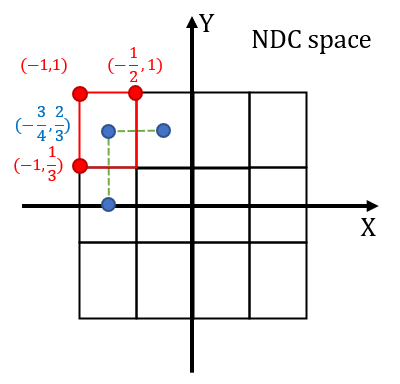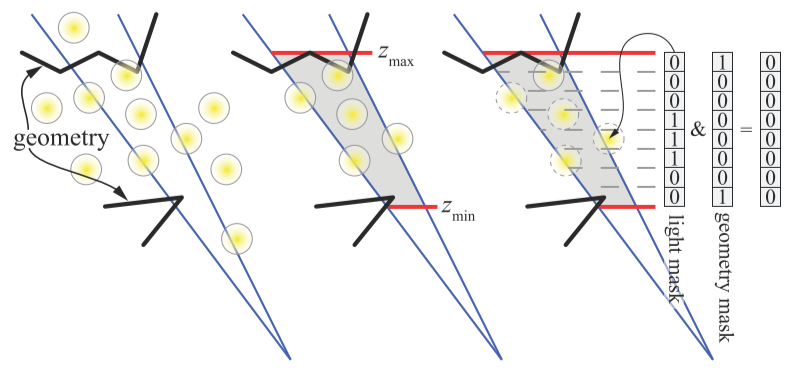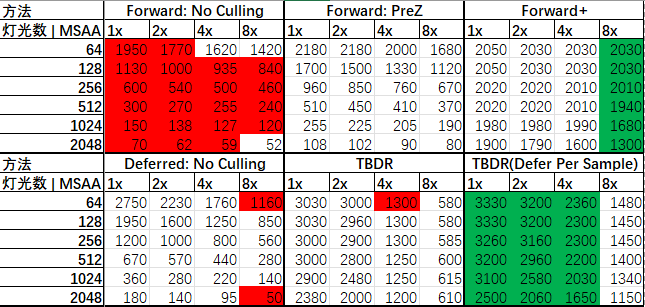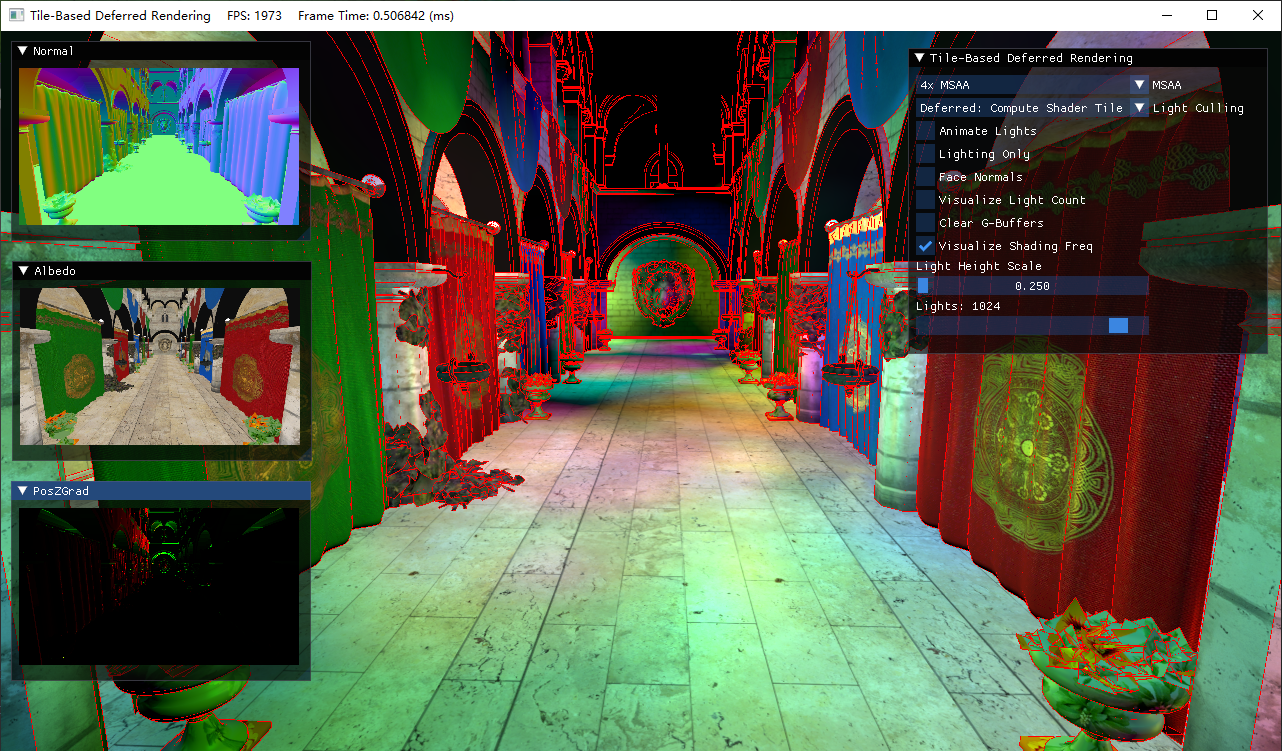# DirectX11 With Windows SDK–37 延迟渲染：光源剔除# 前言

• 计算着色器
• 结构化缓冲区

DirectX11 With Windows SDK完整目录

Github项目源码

# 基于分块(Tile-Based)的光源剔除

## 分块子视锥体深度范围的计算// 确定分块(tile)的大小用于光照去除和相关的权衡取舍

groupshared uint s_MinZ;
groupshared uint s_MaxZ;

// 当前tile的光照列表
groupshared uint s_TileLightIndices[MAX_LIGHTS];
groupshared uint s_TileNumLights;

uint groupIndex : SV_GroupIndex
)
{
//
// 获取表面数据，计算当前分块的视锥体
//

SurfaceData surfaceSamples[MSAA_SAMPLES];
ComputeSurfaceDataFromGBufferAllSamples(globalCoords, surfaceSamples);

// 寻找所有采样中的Z边界
float minZSample = g_CameraNearFar.y;
float maxZSample = g_CameraNearFar.x;
{
[unroll]
for (uint sample = 0; sample < MSAA_SAMPLES; ++sample)
{
// 避免对天空盒或其它非法像素着色
float viewSpaceZ = surfaceSamples[sample].posV.z;
bool validPixel =
viewSpaceZ >= g_CameraNearFar.x &&
viewSpaceZ < g_CameraNearFar.y;
[flatten]
if (validPixel)
{
minZSample = min(minZSample, viewSpaceZ);
maxZSample = max(maxZSample, viewSpaceZ);
}
}
}

// 初始化共享内存中的光照列表和Z边界
if (groupIndex == 0)
{
s_TileNumLights = 0;
s_NumPerSamplePixels = 0;
s_MinZ = 0x7F7FFFFF; // 最大浮点数
s_MaxZ = 0;
}

GroupMemoryBarrierWithGroupSync();

// 注意：这里可以进行并行归约(parallel reduction)的优化，但由于我们使用了MSAA并
// 存储了多重采样的像素在共享内存中，逐渐增加的共享内存压力实际上**减小**内核的总
// 体运行速度。因为即便是在最好的情况下，在目前具有典型分块(tile)大小的的架构上，
// 并行归约的速度优势也是不大的。
// 只有少量实际合法样本的像素在其中。
if (maxZSample >= minZSample)
{
InterlockedMin(s_MinZ, asuint(minZSample));
InterlockedMax(s_MaxZ, asuint(maxZSample));
}

GroupMemoryBarrierWithGroupSync();

float minTileZ = asfloat(s_MinZ);
float maxTileZ = asfloat(s_MaxZ);
float4 frustumPlanes;
ConstructFrustumPlanes(groupId, minTileZ, maxTileZ, frustumPlanes);
// ...
}


## 分块视锥体矩阵的推导及*面的构建

### 推导分块所属视锥体

\begin{aligned} P_{persp}&=P_{persp\rightarrow ortho} P_{ortho} \\ &= \begin{bmatrix} n & 0 & 0 & 0 \\ 0 & n & 0 & 0 \\ 0 & 0 & n + f & 1 \\ 0 & 0 & -fn & 0 \\ \end{bmatrix}\begin{bmatrix} \frac{1}{rn\cdot tan(\alpha/2)} & 0 & 0 & 0 \\ 0 & \frac{1}{n\cdot tan(\alpha/2)} & 0 & 0 \\ 0 & 0 & \frac{1}{f – n} & 0 \\ 0 & 0 & \frac{-n}{f – n} & 1 \\ \end{bmatrix} \\ &= \begin{bmatrix} \frac{1}{rtan(\alpha/2)} & 0 & 0 & 0 \\ 0 & \frac{1}{tan(\alpha/2)} & 0 & 0 \\ 0 & 0 & \frac{f}{f-n} & 1 \\ 0 & 0 & -\frac{nf}{f-n} & 0 \\ \end{bmatrix} \end{aligned}

\begin{aligned} [x,y,z,1]\begin{bmatrix} m_{00} & 0 & 0 & 0 \\ 0 & m_{11} & 0 & 0 \\ 0 & 0 & m_{22} & 1 \\ 0 & 0 & m_{32} & 0 \\ \end{bmatrix}&=[m_{00}x,m_{11}y,m_{22}z+m_{32},z]\\ &\Rightarrow[\frac{m_{00}x}{z},\frac{m_{11}y}{z},m_{22}+\frac{m_{32}}{z}, 1] \end{aligned}\\

\begin{aligned} \begin{bmatrix}x & y & z & 1\end{bmatrix}\begin{bmatrix} s_x\cdot m_{00} & 0 & 0 & 0 \\ 0 & s_y\cdot m_{11} & 0 & 0 \\ t_x & t_y & m_{22} & 1 \\ 0 & 0 & m_{32} & 0 \\ \end{bmatrix}&=[s_x(m_{00}x)+t_x z,\; s_y(m_{11}y)+t_y z,\;m_{22}z+m_{32},\;z]\\ &\Rightarrow[\frac{s_x(m_{00}x)}{z}+t_x\;,\frac{s_y(m_{11}y)}{z}+t_y\;,m_{22}+\frac{m_{32}}{z}\;, 1] \end{aligned}\\$s_x =W/groupDim\\ s_y=H/groupDim$

$t_x=s_x(1-\frac{groupDim}{W})\\ t_y=s_y(-1+\frac{groupDim}{H})$

\begin{aligned} t_x&=s_x(1-\frac{groupDim}{W}-G_x\frac{2\cdot groupDim}{W})\\ &=s_x-1-2G_x\\ t_y&=s_y(-1+\frac{groupDim}{H}-G_y\frac{2\cdot groupDim}{H})\\ &=-s_y+1+2G_y \end{aligned}

### Gribb/Hartmann法提取视锥体*面

$right=col_3-col_0\\ left=col_3+col_0\\ top=col_3-col_1\\ bottom=col_3+col_1\\ near=(0, 0, 1, -minZ)\\ far=(0,0,-1,maxZ)$

• 如果待提取的矩阵为VP，提取出的视锥体*面位于世界空间
• 如果待提取的矩阵为WVP，提取出的视锥体*面位于物体空间

void ConstructFrustumPlanes(uint3 groupId, float minTileZ, float maxTileZ, out float4 frustumPlanes)
{

// 注意：下面的计算每个分块都是统一的(例如：不需要每个线程都执行)，但代价低廉。
// 我们可以只是先为每个分块预计算视锥*面，然后将结果放到一个常量缓冲区中...
// 只有当投影矩阵改变的时候才需要变化，因为我们是在观察空间执行，
// 然后我们就只需要计算*/远*面来贴紧我们实际的几何体。

// 原Intel样例程序计算的Scale和Bias有误，这里重新推导了一遍
float2 tileScale = float2(g_FramebufferDimensions.xy) / COMPUTE_SHADER_TILE_GROUP_DIM;
float2 tileBias = tileScale - 1.0f - 2.0f * float2(groupId.xy);

// 计算当前分块视锥体的投影矩阵
float4 c1 = float4(g_Proj._11 * tileScale.x, 0.0f, tileBias.x, 0.0f);
float4 c2 = float4(0.0f, g_Proj._22 * tileScale.y, -tileBias.y, 0.0f);
float4 c4 = float4(0.0f, 0.0f, 1.0f, 0.0f);

// Gribb/Hartmann法提取视锥体*面
// 侧面
frustumPlanes = c4 - c1; // 右裁剪*面
frustumPlanes = c4 + c1; // 左裁剪*面
frustumPlanes = c4 - c2; // 上裁剪*面
frustumPlanes = c4 + c2; // 下裁剪*面
// */远*面
frustumPlanes = float4(0.0f, 0.0f, 1.0f, -minTileZ);
frustumPlanes = float4(0.0f, 0.0f, -1.0f, maxTileZ);

// 标准化视锥体*面(*/远*面已经标准化)
[unroll]
for (uint i = 0; i < 4; ++i)
{
frustumPlanes[i] *= rcp(length(frustumPlanes[i].xyz));
}

// ...


### Gribb/Hartmann法提取视锥体*面

$right=col_3-col_0\\ left=col_3+col_0\\ top=col_3-col_1\\ bottom=col_3+col_1\\ near=(0, 0, 1, -minZ)\\ far=(0,0,-1,maxZ)$

• 如果待提取的矩阵为VP，提取出的视锥体*面位于世界空间
• 如果待提取的矩阵为WVP，提取出的视锥体*面位于物体空间

void ConstructFrustumPlanes(uint3 groupId, float minTileZ, float maxTileZ, out float4 frustumPlanes)
{

// 注意：下面的计算每个分块都是统一的(例如：不需要每个线程都执行)，但代价低廉。
// 我们可以只是先为每个分块预计算视锥*面，然后将结果放到一个常量缓冲区中...
// 只有当投影矩阵改变的时候才需要变化，因为我们是在观察空间执行，
// 然后我们就只需要计算*/远*面来贴紧我们实际的几何体。

// 从[0, 1]中找出缩放/偏移
float2 tileScale = float2(g_FramebufferDimensions.xy) * rcp(float(2 * COMPUTE_SHADER_TILE_GROUP_DIM));
float2 tileBias = tileScale - float2(groupId.xy);

// 计算当前分块视锥体的投影矩阵
float4 c1 = float4(g_Proj._11 * tileScale.x, 0.0f, tileBias.x, 0.0f);
float4 c2 = float4(0.0f, -g_Proj._22 * tileScale.y, tileBias.y, 0.0f);
float4 c4 = float4(0.0f, 0.0f, 1.0f, 0.0f);

// Gribb/Hartmann法提取视锥体*面
// 侧面
frustumPlanes = c4 - c1; // 右裁剪*面
frustumPlanes = c4 + c1; // 左裁剪*面
frustumPlanes = c4 - c2; // 上裁剪*面
frustumPlanes = c4 + c2; // 下裁剪*面
// */远*面
frustumPlanes = float4(0.0f, 0.0f, 1.0f, -minTileZ);
frustumPlanes = float4(0.0f, 0.0f, -1.0f, maxTileZ);

// 标准化视锥体*面(*/远*面已经标准化)
[unroll]
for (uint i = 0; i < 4; ++i)
{
frustumPlanes[i] *= rcp(length(frustumPlanes[i].xyz));
}

// ...


## 光源剔除

    //
// 对当前分块(tile)进行光照剔除
//

uint totalLights, dummy;
g_Light.GetDimensions(totalLights, dummy);

// 组内每个线程承担一部分光源的碰撞检测计算
for (uint lightIndex = groupIndex; lightIndex < totalLights; lightIndex += COMPUTE_SHADER_TILE_GROUP_SIZE)
{
PointLight light = g_Light[lightIndex];

// 点光源球体与tile视锥体*面的相交测试
// 当球心位于*面外侧且距离超过r，则没有相交
bool inFrustum = true;
[unroll]
for (uint i = 0; i < 6; ++i)
{
float d = dot(frustumPlanes[i], float4(light.posV, 1.0f));
inFrustum = inFrustum && (d >= -light.attenuationEnd);
}

[branch]
if (inFrustum)
{
// 将光照追加到列表中
uint listIndex;
s_TileLightIndices[listIndex] = lightIndex;
}
}

GroupMemoryBarrierWithGroupSync();


## 在计算着色器完成光照阶段

RWStructuredBuffer<uint2> g_Framebuffer : register(u1);

// ...

// 当前tile中需要逐样本着色的像素列表
// 我们将两个16位x/y坐标编码进一个uint来节省共享内存空间
groupshared uint s_NumPerSamplePixels;

//--------------------------------------------------------------------------------------
// 用于写入我们的1D MSAA UAV
void WriteSample(uint2 coords, uint sampleIndex, float4 value)
{
}

// 将两个<=16位的坐标值打包进单个uint
uint PackCoords(uint2 coords)
{
return coords.y << 16 | coords.x;
}
// 将单个uint解包成两个<=16位的坐标值
uint2 UnpackCoords(uint coords)
{
return uint2(coords & 0xFFFF, coords >> 16);
}

uint groupIndex : SV_GroupIndex
)
{

// ...

//
// 光照阶段。只处理在屏幕区域的像素(单个分块可能超出屏幕边缘)
//
uint numLights = s_TileNumLights;
if (all(globalCoords < g_FramebufferDimensions.xy))
{
[branch]
if (numLights > 0)
{

float3 lit = float3(0.0f, 0.0f, 0.0f);
for (uint tileLightIndex = 0; tileLightIndex < numLights; ++tileLightIndex)
{
PointLight light = g_Light[s_TileLightIndices[tileLightIndex]];
AccumulateColor(surfaceSamples, light, lit);
}

// 计算样本0的结果
WriteSample(globalCoords, 0, float4(lit, 1.0f));

[branch]
{
// 创建需要进行逐样本着色的像素列表,延迟其余样本的着色
uint listIndex;
s_PerSamplePixels[listIndex] = PackCoords(globalCoords);
}
else
{
// 否则进行逐像素着色，将样本0的结果也复制到其它样本上
[unroll]
for (uint sample = 1; sample < MSAA_SAMPLES; ++sample)
{
WriteSample(globalCoords, sample, float4(lit, 1.0f));
}
}
}
else
{
// 没有光照的影响，清空所有样本
[unroll]
for (uint sample = 0; sample < MSAA_SAMPLES; ++sample)
{
WriteSample(globalCoords, sample, float4(0.0f, 0.0f, 0.0f, 0.0f));
}
}
}

#if MSAA_SAMPLES > 1
GroupMemoryBarrierWithGroupSync();

// 现在处理那些需要逐样本着色的像素
// 注意：每个像素需要额外的MSAA_SAMPLES - 1次着色passes
const uint shadingPassesPerPixel = MSAA_SAMPLES - 1;
uint globalSamples = s_NumPerSamplePixels * shadingPassesPerPixel;

for (uint globalSample = groupIndex; globalSample < globalSamples; globalSample += COMPUTE_SHADER_TILE_GROUP_SIZE) {
uint listIndex = globalSample / shadingPassesPerPixel;
uint sampleIndex = globalSample % shadingPassesPerPixel + 1;        // 样本0已经被处理过了

uint2 sampleCoords = UnpackCoords(s_PerSamplePixels[listIndex]);
SurfaceData surface = ComputeSurfaceDataFromGBufferSample(sampleCoords, sampleIndex);

float3 lit = float3(0.0f, 0.0f, 0.0f);
for (uint tileLightIndex = 0; tileLightIndex < numLights; ++tileLightIndex) {
PointLight light = g_Light[s_TileLightIndices[tileLightIndex]];
AccumulateColor(surface, light, lit);
}
WriteSample(sampleCoords, sampleIndex, float4(lit, 1.0f));
}
#endif
}


## 分块光源剔除的优缺点

• 对含有大量动态光源的场景，能够有效减少相当部分无关光源的计算
• 对延迟渲染而言，光源的剔除和计算可以同时在计算着色器中进行

• tile的光源列表信息受动态光源、摄像机变换的影响，需要每帧都重新计算一次，带来一定的开销
• 基于屏幕空间tile的划分仍不够精细，没有考虑到深度值的划分
• 需要支持计算着色器

• 分块2.5D光源剔除
• Cluster Light Culling（分簇光源剔除）

## 分块2.5D光源剔除2.5D光源剔除的具体做法为：

• 使用16×16大小的Tile先进行子视锥体与光源的碰撞测试，生成一个初步的光源列表
• 然后以64个线程为一组，每个线程对Tile中的4个像素与光源列表的光进行比较，进一步剔除这个列表。如果当前Tile中没有一个像素的位置在点光源内，那么该灯光就可以从列表中剔除。由此产生的灯光集可以算是相当准确的，因为只有那些保证至少影响一个像素的灯光被保存。

# Forward+

• Pre-Z Pass，因为在光源剔除阶段我们需要利用深度信息，所以记录场景深度信息到深度缓冲区中
• 执行Tile-Based Light Culling
• 前向渲染，仅绘制和深度缓冲区深度值相等的像素，并利用所在tile的光源列表来计算颜色

struct TileInfo
{
uint tileNumLights;
uint tileLightIndices[MAX_LIGHT_INDICES];
};

RWStructuredBuffer<TileInfo> g_TilebufferRW : register(u0);

groupshared uint s_MinZ;
groupshared uint s_MaxZ;

// 当前tile的光照列表
groupshared uint s_TileLightIndices[MAX_LIGHTS >> 3];
groupshared uint s_TileNumLights;

// 当前tile中需要逐样本着色的像素列表
// 我们将两个16位x/y坐标编码进一个uint来节省共享内存空间
groupshared uint s_NumPerSamplePixels;

uint groupIndex : SV_GroupIndex
)
{
//
// 获取深度数据，计算当前分块的视锥体
//

// 寻找所有采样中的Z边界
float minZSample = g_CameraNearFar.y;
float maxZSample = g_CameraNearFar.x;
{
[unroll]
for (uint sample = 0; sample < MSAA_SAMPLES; ++sample)
{
// 这里取的是深度缓冲区的Z值
float viewSpaceZ = g_Proj._m32 / (zBuffer - g_Proj._m22);

// 避免对天空盒或其它非法像素着色
bool validPixel =
viewSpaceZ >= g_CameraNearFar.x &&
viewSpaceZ < g_CameraNearFar.y;
[flatten]
if (validPixel)
{
minZSample = min(minZSample, viewSpaceZ);
maxZSample = max(maxZSample, viewSpaceZ);
}
}
}

// 初始化共享内存中的光照列表和Z边界
if (groupIndex == 0)
{
s_TileNumLights = 0;
s_NumPerSamplePixels = 0;
s_MinZ = 0x7F7FFFFF; // 最大浮点数
s_MaxZ = 0;
}

GroupMemoryBarrierWithGroupSync();

// 注意：这里可以进行并行归约(parallel reduction)的优化，但由于我们使用了MSAA并
// 存储了多重采样的像素在共享内存中，逐渐增加的共享内存压力实际上**减小**内核的总
// 体运行速度。因为即便是在最好的情况下，在目前具有典型分块(tile)大小的的架构上，
// 并行归约的速度优势也是不大的。
// 只有少量实际合法样本的像素在其中。
if (maxZSample >= minZSample)
{
InterlockedMin(s_MinZ, asuint(minZSample));
InterlockedMax(s_MaxZ, asuint(maxZSample));
}

GroupMemoryBarrierWithGroupSync();

float minTileZ = asfloat(s_MinZ);
float maxTileZ = asfloat(s_MaxZ);
float4 frustumPlanes;
ConstructFrustumPlanes(groupId, minTileZ, maxTileZ, frustumPlanes);

//
// 对当前分块(tile)进行光照剔除
//

uint totalLights, dummy;
g_Light.GetDimensions(totalLights, dummy);

// 计算当前tile在光照索引缓冲区中的位置
uint tilebufferIndex = groupId.y * dispatchWidth + groupId.x;

// 组内每个线程承担一部分光源的碰撞检测计算
[loop]
for (uint lightIndex = groupIndex; lightIndex < totalLights; lightIndex += COMPUTE_SHADER_TILE_GROUP_SIZE)
{
PointLight light = g_Light[lightIndex];

// 点光源球体与tile视锥体的碰撞检测
bool inFrustum = true;
[unroll]
for (uint i = 0; i < 6; ++i)
{
float d = dot(frustumPlanes[i], float4(light.posV, 1.0f));
inFrustum = inFrustum && (d >= -light.attenuationEnd);
}

[branch]
if (inFrustum)
{
// 将光照追加到列表中
uint listIndex;
g_TilebufferRW[tilebufferIndex].tileLightIndices[listIndex] = lightIndex;
}
}

GroupMemoryBarrierWithGroupSync();

if (groupIndex == 0)
{
g_TilebufferRW[tilebufferIndex].tileNumLights = s_TileNumLights;
}
}


StructuredBuffer<TileInfo> g_Tilebuffer : register(t9);
//--------------------------------------------------------------------------------------
// 计算点光源着色
float4 ForwardPlusPS(VertexPosHVNormalVTex input) : SV_Target
{
// 计算当前像素所属的tile在光照索引缓冲区中的位置
uint tilebufferIndex = (uint) input.posH.y / COMPUTE_SHADER_TILE_GROUP_DIM * dispatchWidth +

float3 litColor = float3(0.0f, 0.0f, 0.0f);
uint numLights = g_Tilebuffer[tilebufferIndex].tileNumLights;
[branch]
if (g_VisualizeLightCount)
{
litColor = (float(numLights) * rcp(255.0f)).xxx;
}
else
{
SurfaceData surface = ComputeSurfaceDataFromGeometry(input);
for (uint lightIndex = 0; lightIndex < numLights; ++lightIndex)
{
PointLight light = g_Light[g_Tilebuffer[tilebufferIndex].tileLightIndices[lightIndex]];
AccumulateColor(surface, light, litColor);
}
}

return float4(litColor, 1.0f);
}


## Forward+的优缺点

• 由于结合了分块光源剔除，使得前向渲染的效率能够得到有效提升
• 强制Pre-Z Pass可以过滤掉大量不需要执行的像素片元
• 前向渲染对材质的支持也比较简单
• 降低了带宽占用
• 支持透明物体绘制
• 支持硬件MSAA

• 相比于延迟渲染的执行效率还是会慢一些
• 需要支持计算着色器

# 性能对比• 将分支中非0样本着色的过程推迟，先将哪些需要逐样本着色的像素添加到像素列表中
• 完成0号样本的着色后，Tile中所有线程分担这些需要逐样本计算的像素着色

# 演示• MSAA：默认关闭，可选2x、4x、8x
• 光照剔除模式：默认开启延迟渲染+无光照剔除，可选前向渲染、带Pre-Z Pass的前向渲染
• Animate Lights：灯光的移动
• Face Normals：使用面法线
• Clear G-Buffer：默认不需要清除G-Buffer再来绘制，该选项开启便于观察G-Buffer中的图
• Visualize Light Count：可视化每个像素渲染的光照数，最大255。
• Light Height Scale：将所有的灯光按一定比例抬高
• Lights：灯光数，2的指数幂
• Normal图：展示了从Normal_Specular G-Buffer还原出的世界坐标下的法线，经[-1, 1]到[0, 1]的映射
• Albedo图：展示了Albedo G-Buffer# 练习题

1. 尝试实现分块2.5D光源剔除

# 补充&参考

DirectX11 With Windows SDK完整目录

Github项目源码

2 total views,  1 views today